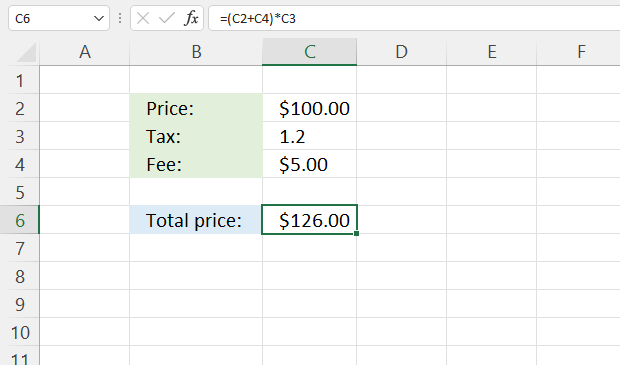Author: Oscar Cronquist Article last updated on September 29, 2022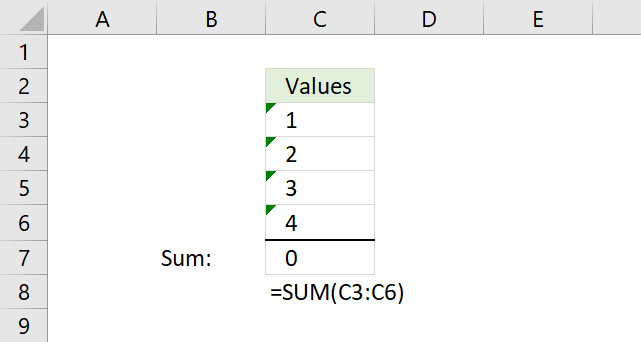This article explains why your formula is not working properly, there are usually four different things that can go wrong.

## 1. Formula not working - check the function arguments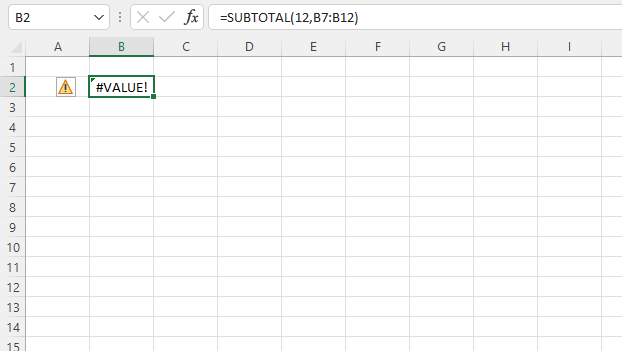This example shows a function in cell B2 that returns a #VALUE! error, this may happen when there is something wrong with the arguments.

The SUBTOTAL function allows you to use a number between 1 and 11, the first argument is in this example 12 which is an invalid argument.

How to quickly show function arguments?

Type the function in a cell, and then the beginning parentheses, Excel instantly shows the arguments and in some cases, allowed values.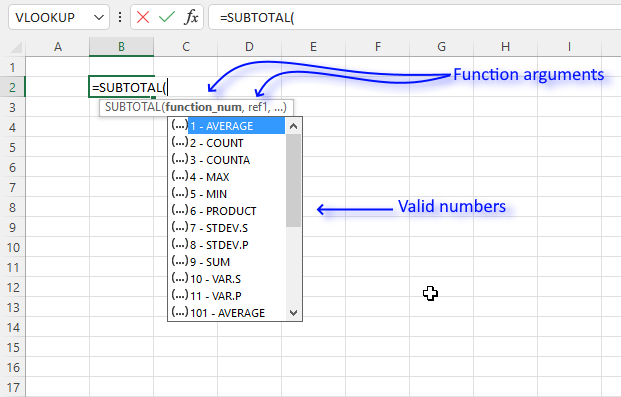Here is a list of Excel functions and their arguments: Excel functions

Here is a list of common errors: Excel formula errors

## 2. How to solve "numbers stored as text"?The first one is numbers stored as text, demonstrated in the picture above.

Why is the SUM function in cell C7 returning 0 (zero) in the picture above?
The numbers in cell range C3:C6 are stored as text. You can see that by the green arrow in each cell's top left corner.

Why is this happening?
The cell range C3:C6 was formatted as text cells before you entered the numbers in these cells.

How to solve it?
Select cell range C3:C6, and press with left mouse button on the exclamation mark symbol (or exclamation point if you are American) to open a menu.
Press with mouse on "Convert to Number", the green arrows disappear and the SUM function formula works as expected again.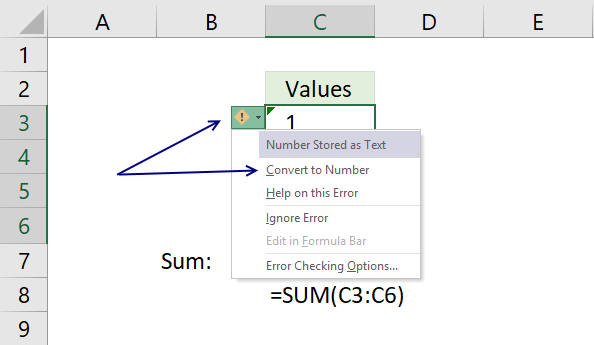## 3. How to change the "manual calculation" setting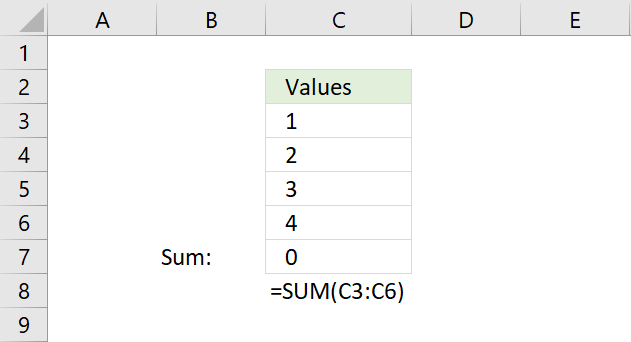The SUM formula in cell C7 returns 0 (zero), why is this happening?

Check if your workbook is in manual calculation mode. Go to tab "Formulas" on the ribbon, then press with left mouse button on the "Calculations Options" button.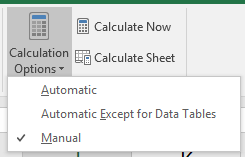In this case, the setting was on "Manual", changing it back to "Automatic" makes the SUM formula work as intended again.

## 4. The cell shows the formula and not the result - here is how to fix it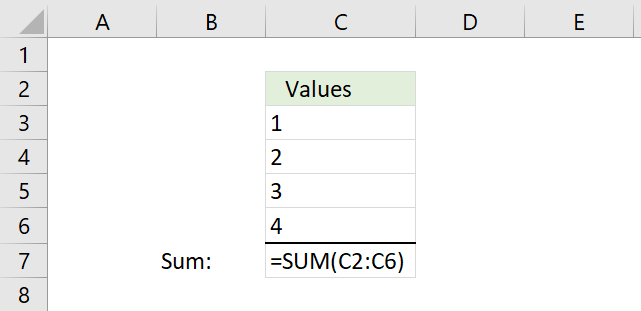Cell C7 displays the formula, not the result. Why is this happening?

Go to tab "Formulas", check if "Show Formulas" button is enabled (highlighted).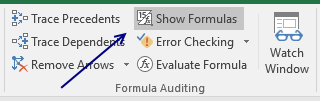Press with mouse on the "Show Formulas" button to disable it. The cell now shows the result of the SUM calculation. If this is not working, read section 5 below.

## 5. Formula cell formatted as textThe cell in C7 shows the formula? I don't want that, I want to see the calculation result.

Select cell C7, press CTRL + 1 on your keyboard.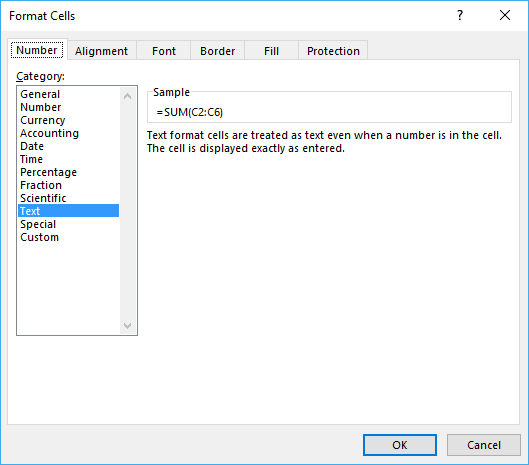This reveals that the cell is formatted as "Text" making it show the formula and not the result. Press with mouse on "General" and then on the OK button.

Now it shows the output from the formula.

## 6. Formula not working - #NAME? error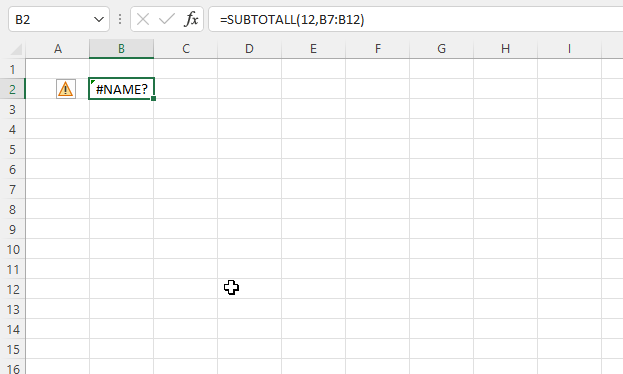The #NAME? error hints that you misspelled the function name.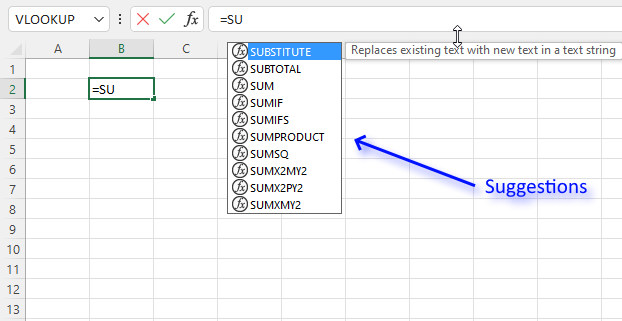Tip! Check the suggestions while typing the formula. Use the arrow up and down keys to change the selected suggestion. Use the TAB key to auto-complete the selected suggestion.

## 7. How to troubleshoot a formula not working as intended?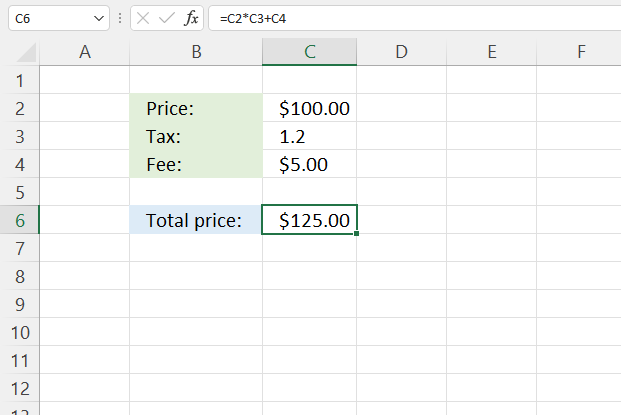The image above shows a formula in cell C6 that returns no error, however, the calculation is wrong. How do we troubleshoot this formula to find what is wrong in the calculation?

The "Evaluate formula" tool lets us see the calculation steps in greater detail. Here is how to start the tool:

1. Select the cell you want to troubleshoot, in this example cell C6.
2. Go to the tab "Formulas" on the ribbon.
3. Press with left mouse button on the "Evaluate Formula" button located on the ribbon.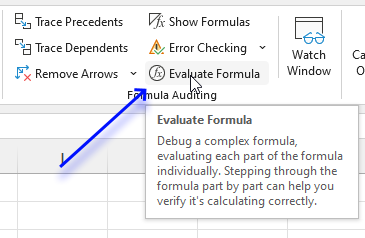4. A dialog box appears.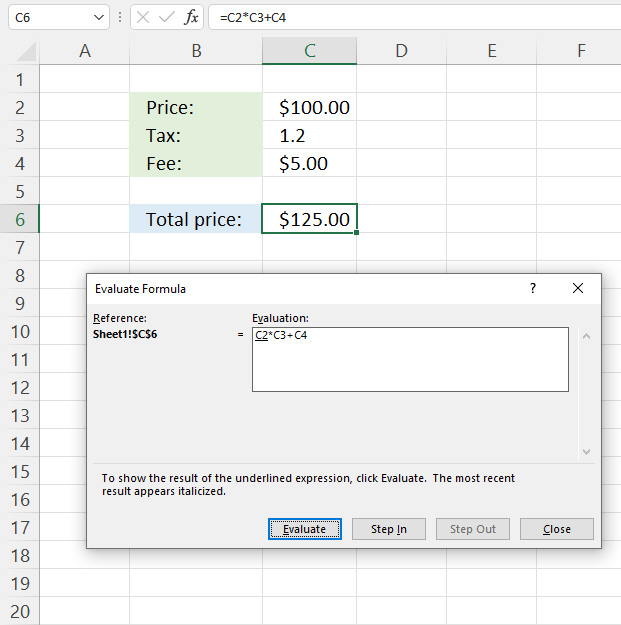The dialog box shows the formula in the white box and has four buttons below. The underlined expression shows what part of the formula will be calculated in the next step.

The italicized text is the most recent result, press with left mouse button on the "Evaluate" button to start the formula evaluation.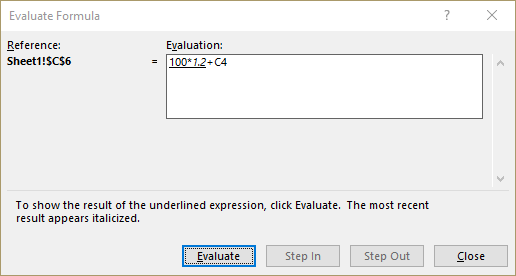Keep press with left mouse button oning on the "Evaluate" button until greyed out to see all steps in the calculation, this helps to identify where the problem is.

The tool shows that the formula multiples values in cells C2 and C3 before adding the number in cell C4. We need to add C2 with C4 and then multiply with C3.

Press with left mouse button on the "Close" button to dismiss the dialog box.

The parentheses allow us to control the order of operation. Here is the final formula:

=(C2+C4)*C3